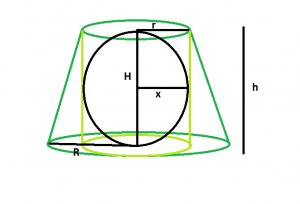# Largest sphere that can be inscribed in a right circular cylinder inscribed in a frustum

• Last Updated : 25 Sep, 2022

Given here is a frustum of height h, top-radius r & base-radius R, which inscribes a right circular cylinder which in turn inscribes a sphere . The task is to find the largest possible volume of this sphere.

Examples:

Input: r = 5, R = 8, h = 11
Output: 523.333

Input: r = 9, R = 14, h = 20
Output:3052.08Approach: Let the height of the cylinder = H, radius of the sphere = x
We know, the height and radius of the cylinder inscribed within the frustum is equal to the height and top-radius of the frustum respectively(Please refer here).So the height of the cylinder = h, radius of the cylinder = r
Also, radius of the sphere inscribed within a cylinder is equal to radius of the cylinder(Please refer here), so x = r
So, volume of the sphere, V = 4*π*r^3/3.

Below is the implementation of the above approach:

## C++

 `// C++ Program to find the biggest sphere``// that can be inscribed within a right``// circular cylinder which in turn is inscribed``// within a frustum``#include ``using` `namespace` `std;` `// Function to find the biggest sphere``float` `sph(``float` `r, ``float` `R, ``float` `h)``{` `    ``// the radii and height cannot be negative``    ``if` `(r < 0 && R < 0 && h < 0)``        ``return` `-1;` `    ``// radius of the sphere``    ``float` `x = r;` `    ``// volume of the sphere``    ``float` `V = (4 * 3.14 * ``pow``(r, 3)) / 3;` `    ``return` `V;``}` `// Driver code``int` `main()``{``    ``float` `r = 5, R = 8, h = 11;``    ``cout << sph(r, R, h) << endl;` `    ``return` `0;``}`

## Java

 `// Java Program to find the biggest sphere``// that can be inscribed within a right``// circular cylinder which in turn is inscribed``// within a frustum``import` `java.lang.Math;` `class` `gfg``{``    ` `// Function to find the biggest sphere``static` `float` `sph(``float` `r, ``float` `R, ``float` `h)``{` `    ``// the radii and height cannot be negative``    ``if` `(r < ``0` `&& R < ``0` `&& h < ``0``)``        ``return` `-``1``;` `    ``// radius of the sphere``    ``float` `x = r;` `    ``// volume of the sphere``    ``float` `V = (``float``)(``4` `* ``3``.14f * Math.pow(r, ``3``)) / ``3``;` `    ``return` `V;``}` `// Driver code``public` `static` `void` `main(String[] args)``{``    ``float` `r = ``5``, R = ``8``, h = ``11``;``    ``System.out.println(sph(r, R, h));``}``}` `// This Code is contributed by Code_Mech.`

## Python3

 `# Python3 Program to find the biggest sphere``# that can be inscribed within a right``# circular cylinder which in turn is inscribed``# within a frustum``import` `math as mt` `# Function to find the biggest sphere``def` `sph(r, R, h):` `    ``# the radii and height cannot``    ``# be negative``    ``if` `(r < ``0` `and` `R < ``0` `and` `h < ``0``):``        ``return` `-``1` `    ``# radius of the sphere``    ``x ``=` `r` `    ``# volume of the sphere``    ``V ``=` `(``4` `*` `3.14` `*` `pow``(r, ``3``)) ``/` `3` `    ``return` `V` `# Driver code``r, R, h ``=` `5``, ``8``, ``11``print``(sph(r, R, h))` `# This code is contributed by``# Mohit kumar 29`

## C#

 `// C# Program to find the biggest sphere``// that can be inscribed within a right``// circular cylinder which in turn is``// inscribed within a frustum``using` `System;` `class` `gfg``{``    ` `    ``// Function to find the biggest sphere``    ``static` `float` `sph(``float` `r, ``float` `R, ``float` `h)``    ``{``    ` `        ``// the radii and height``        ``// cannot be negative``        ``if` `(r < 0 && R < 0 && h < 0)``            ``return` `-1;``    ` `        ``// radius of the sphere``        ``float` `x = r;``    ` `        ``// volume of the sphere``        ``float` `V = (``float``)(4 * 3.14f *``                    ``Math.Pow(r, 3)) / 3;``    ` `        ``return` `V;``    ``}``    ` `    ``// Driver code``    ``public` `static` `void` `Main()``    ``{``        ``float` `r = 5, R = 8, h = 11;``        ``Console.WriteLine(sph(r, R, h));``    ``}``}` `// This code is contributed by Ryuga`

## PHP

 ``

## Javascript

 ``

Output:

`523.333`

Time Complexity: O(1)
Auxiliary Space: O(1)

My Personal Notes arrow_drop_up